Euler characteristic and genus

We now want to give the precise definition of genus. We can start with the famous formula of Euler. Given a polyhedronwith V vertices, E edges and F faces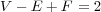The unstated assumption is that the surface of the polyhedron is homeomorphic to the sphere. However, we can form polyhedra homeomorphic to other surfaces. The following is homeomorphic to a torus,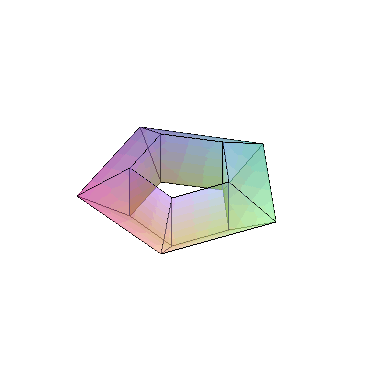In such cases, we get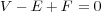The point is that this expression called the Euler characteristic χ depends only on the surface. If S is compact oriented surface (e.g. a complex curves with points of infinity included) then χ(S) is known to even, and the genus g can be defined by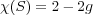The genus can be understood intuitively as the “number of holes”. For example, a genus two surface or “two holed doughnut” is depicted below.Consider the hyperelliptic curve X defined by an equation y2 = p(x) where p(x) has an odd degree, say n, with distinct roots. We give a formula (due to Riemann and Hurwitz) for the genus g of X. We triangulate C ∪{∞} so that the roots of p(x) and are included among the vertices. Let’s say that there are V verticies, E edges and F faces. So that V - E + F = 2. The preimage of this triangulation gives a triangulation of X with V vertices etcetera. We have V ′- E+ F= 2 - 2g. The map from X C ∪{∞} is two to one except at vertices lying over the roots and where it one to one. Thus V = 2V - n - 1, E= 2E, F= 2F, so that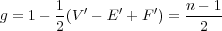Although the definition is topological, it turns out that the genus is strongly connected to the complex function theory of a curve. The relationship is given by the Riemann-Roch theorem. We will explain a special case. First observe that a holomorphic function on a compact connected complex curve X is necessarily constant.

Proof: Since X is compact, the modulus of the function must attain a maximum at some point p. By the maximum modulus principle the function must be constant in a neighbourhood of p. This implies that f = f(p) everywhere since the difference has nonisolated zero.

Therefore nonconstant meromorphic functions on X must have singularities. Which raises the question, what are simplest possible singularities? Choose points p1,pk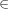X and positive integers n1,nk. Then the Riemann-Roch theorem tells us that there is a nonconstant function with poles only at pi of order at most ni, provided that n1 + + nk > g. A standard proof uses a refined form of the Euler characteristic.